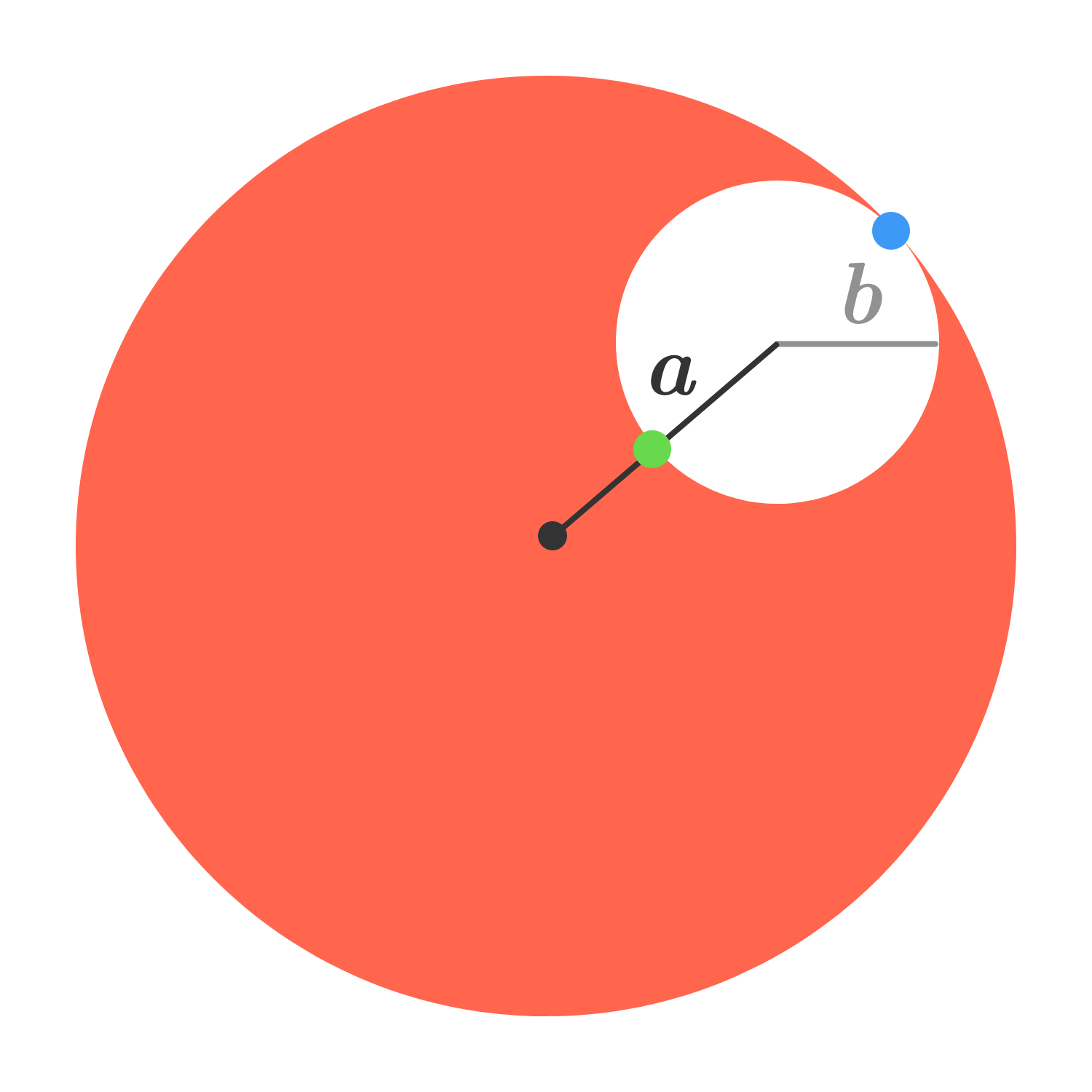# Holed onA sphere of uniform mass density $\rho$ and radius $a+b$ has a spherical hole of radius $b$ drilled a distance $a$ from its center. The gravitational field is measured at the far side of the hole (at the blue point) and is found to be $g_0.$

What will the field measure at the near side of the hole (at the green point)?

×